# New Keyboard Image

This entry was posted in fcitx development. Bookmark the permalink.

### 10 Responses to New Keyboard Image

1.依云 says: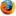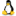很漂亮。不过，点到中间的空白处会不会有响应呢？

2.Enih says: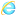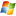有点写实了，越抽象越好。比如，中间的空白处造成不必要的计算浪费和用户的好奇心。

3.右京样一 says: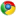空白处就是移动窗口嘛……

有种QtCurve的感觉，比原来的漂亮多了……
貌似Shift那个上箭头有点太长了？我键盘上是横宽的，纵向距离很短。其实箭头都不必做得特别大。

4.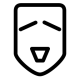csslayer says:@Enih fcitx那个软键盘就是一张贴图而已，所以不论我怎么改图片都不会影响性能的。

顶部的空白是有文字显示的。

5.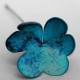kikyous says:只是做了一张图片吗?

6.右京样一 says:@kikyous 人家都说了有顶部文字显示嘛……

7.百里 says:@csslayer
就是说这软键盘可以随便美化么……

8.marseille says:我想使用Control+\调用fcitx，应该怎么办?

9.litkt says: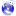vk.conf里好象(因为我搞不清是原有的，还是我自己改乱的)有个小bug，就是数学符号一节里有两个9而没有8.
另我对vk.conf所做的修改，不知对别人来说能否带来更多方便
``` [VK] NAME=希腊字母 w=ω Ω e=ε Ε r=ρ Ρ t=τ Τ y=υ Υ i=ι Ι o=ο Ο p=π Π a=α Α s=σ Σ d=δ Δ f=φ Φ g=γ Γ h=η Η j=θ Θ k=κ Κ l=λ Λ z=ζ Ζ x=χ Χ c=ξ Ξ v=ψ Ψ b=β Β n=ν Ν m=μ Μ```

``` ```

```[VK] NAME=数字序号 1=Ⅰ 2=Ⅱ 3=Ⅲ 4=Ⅳ 5=Ⅴ 6=Ⅵ 7=Ⅶ 8=Ⅷ 9=Ⅸ 0=Ⅹ -=Ⅺ ==Ⅻ ```
差不多就这些。

10.csslayer says:@litkt 非常感谢！数字部分我没有意见，希腊字符部分也许最好按照希腊字母的键盘布局？

下面是我修改后希腊字母部分的布局。会合并到新版本里面的 🙂
w=ς Σ
e=ε Ε
r=ρ Ρ
t=τ Τ
y=υ Υ
u=θ Θ
i=ι Ι
o=ο Ο
p=π Π
a=α Α
s=σ Σ
d=δ Δ
f=φ Φ
g=γ Γ
h=η Η
j=ξ Ξ
k=κ Κ
l=λ Λ
z=ζ Ζ
x=χ Χ
c=ψ Ψ
v=ω Ω
b=β Β
n=ν Ν
m=μ Μ

This site uses Akismet to reduce spam. Learn how your comment data is processed.